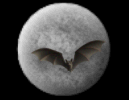God & Science Forum Message Forums: Atm · Astrophotography · Blackholes · Blackholes2 · CCD · Celestron · Domes · Education Eyepieces · Meade · Misc. · God and Science · SETI · Software · UFO · XEphemBe the first pioneers to continue the Astronomy Discussions at our new Astronomy meeting place...The Space and Astronomy Agora Additional Note Forum List | Follow Ups | Post Message | Back to Thread Topics | In Response ToPosted by Alan on January 20, 2004 02:59:27 UTC

I thought I posted this around the time of the above post but maybe there was a hiccup. This is a bit out of date in that I have written stuff since which I haven't typed but for the record here is this note:

Quote: "No, that is not the definition of a parametric representation of a line. In a parametric representation of a line, a parameter is used to label a specific point in the line."

Quote: "Fundamentally, it makes no difference how that parameter is laid out so long as a specific value of the parameter refers to a single point on that line. In a parametric representation of the line, the specific value of the coordinates of each and every point in the line can be represented by functions of that parameter."

"Function" = "way of grouping things".

"Labelling specific points" means distinguishing them apart (as: "specific").

So in a parametric representation of a line you effectively say that the points can be distinguished apart (thus defining the line as a line not a scattered muddle) by ways of grouping things that correspond to "virtual points" (i.e. specific values) of a "parameter".

So you have a line and an "imaginary line" which are connected one-to-one via ways of grouping (making functions with) the "imaginary points" (the specific values) of the parameter?

Since the points on each line (real and imaginary) are specific; the connecting "functions" must be made of precisely synchronised ways of grouping correspondences between line-points and parameter-values (imaginary line points).

If not precisely synchronised, you could get two line points for one parameter-value.

So enough complimentary functions must be present to weed out all unwanted double-assigned labels of points by parameter values; and enough complimentary functions to bring back any lost points from functions that missed some points.

So you end out with a possibly complicated network of connections between three sets:

(1) points on a line;

(2) points (values) in a parameter;

(3) unique connections between them (unique once all connections are seen at once so various connections (functions) can cancel or reinforce each other to get a final meta-function to describe the line (final meta-function: a "space" where the definition of "parameter" collides with the definition of "function".)

Taken together, all these functions do not explain anything about the line and "know" nothing about it other than the complex juggling required among the functions to "see" the line?

The values of the line are in specific correspondences to the values of the parameter via functions of the parameter.

Take out "functions of parameter" and you just have a linear superposition of lines?

(But you could twist the geometry so much that "functions of parameter" was laid out as a line; but "points on a line" now looked scattered all over the place; and "values of parameter" looked scattered all over the place?)

Or you could twist the background geometry a bit, make everything look scattered but yet still be complimentary as:

(1) geometry (imaginary line in background space);

(2) line;

(3) function-processing-of-parameters (an imaginary line say, in "function-space);

(4) values of parameter (imaginary line in parameter value space).

Note I just short-circuited this!

Right here I have x,y,z,t in "p":

I have three imaginary spaces I can call x,y,z;

I have time as "self-referent reference" as "line" defined by "line conserved" (so defined as linear) (by seeing it as at least two meetings among the three imaginary spaces) (so self-refering via the reference of 3 spaces) so one unit of space in "line" defines its beginning and ending in 3 spaces giving the 3 spaces as "reference" to the line's "self-reference" giving space-time singularity.

Any complexity due to "p" (function processing of parameters) would cause interference among the three spaces; this would complexify the space-time definition of this "line"?

So you get a frozen perspective on the line: the parameter is effectively a way of looking at the geometry backgrounding the line from the LINE'S point of view (its inertial reference frame)?

So it is what I said still; a pre-defining characteristic; like the geometry.

We have no priveleged reference frame on the line other than the line itself looking at itself (so have general relativity superposed with special relativity) giving quantum mechanics (the internal configurations of synchronised definitions of math (counting), space (room between two or more), and time (referent self reference)?

Against an asumed fixed geometry:

Juggling of "parameter" (by "functions") allows parameter values to map line values.

The line is defined in its geometry by "parameter functions". Is it defined in its "parameter functions" by geometry? There is the possibility of exchanges between these two backgrounds; of other dimensions to them?

So I just needed to use "parameter functions" as my phrase in saying "p" was a pre-set defining characteristic of the line?

Regards,

Alan